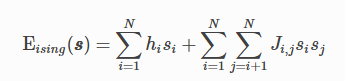# What is the Ising Model?

The Ising model is traditionally used in statistical mechanics. Variables are “spin up” () and “spin down” (), states that correspond to +1 and −1 values. Relationships between the spins, represented by couplings, are correlations or anti-correlations. The objective function expressed as an Ising model is as follows:where the linear coefficients corresponding to qubit biases are h_i, and the quadratic coefficients corresponding to coupling strengths are J_i,j.